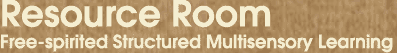Home > Math > Teaching Addition of Fractions with Different Denominators

## Teaching the Addition of Fractions with Different Denominators

Here's something I came up with for adding (or subtracting) fractions with different denominators.

Whereas, almost all students remember how to change a mixed number to an improper fraction

Whereas, many fewer remember how to change fractions to ones with common denominators

I decided to teach common denominators using that same "motor memory" idea. ( more on how and why.)

To wit:

First [this step especially important for students who work better with words], ask: what is this problem asking me to do? ADding fractions. What do you need to add fractions? You need a common denominator.

Second (optional) : rewrite the problem vertically (This will work All The TIme, even for borrowing or other horrifying things, and it doesn't look *anything* like multiplying). To wit:Step three: figure out the common denominator. (whatever way you've taught to do this)

Step four: write the fractions with *just* the fraction bar and the denominator, because that's the part you know. [another common theme of mine is "write what you know, then look for the rest"] Write it right next to the fraction that it is exactly the same thing as (and just do a better job of lining them up than my neophytic images do :-)).Step five: Set up the new fractions.

Figure out what you have to multiply by to get the new denominator (even if it's one, for my very procedural students). Write "x 2" on the bottom, and RIGHT AWAY, write "x2" on the top. Then go to the next fraction. Some students will include "x 1" in the routine; I tell them it's not necessary and why but procedure likers will follow the procedure. (Often, at this point, students will take some ownership of the exact sequence; if so, more power to 'em. If not, I teach them to do all the set ups and then fill in all the numbers.)Step Six: Change the fractions. Follow your very own directions. 2 x 2 = 4, 1 x 2 = 2. (Say it out loud, especially if you're an auditory learner; the visual-kinesthetics will benefit from moving across from one fraction to the next. Since you're multiplying by the same thing, they'll sort of rhyme.)Step seven: add those blighters, same way you added fractions with like denominators before.(Step eight: reduce.)